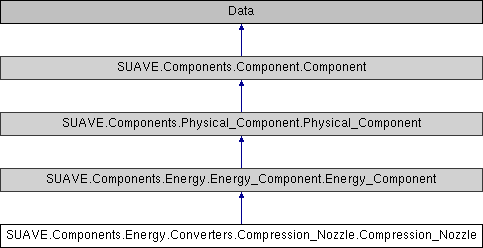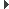SUAVE  2.5.2 An Aerospace Vehicle Environment for Designing Future AircraftSUAVE.Components.Energy.Converters.Compression_Nozzle.Compression_Nozzle Class Reference
Inheritance diagram for SUAVE.Components.Energy.Converters.Compression_Nozzle.Compression_Nozzle:## Public Member Functions

def __defaults__ (self)

def compute (self, conditions)

def compute_scramjet (self, conditions)

## Public Attributes

tag

polytropic_efficiency

pressure_ratio

pressure_recovery

compressibility_effects

compression_levels

thetaPublic Attributes inherited from SUAVE.Components.Energy.Energy_Component.Energy_Component
inputs

outputsPublic Attributes inherited from SUAVE.Components.Physical_Component.Physical_Component
tag

mass_properties

origin

symmetricPublic Attributes inherited from SUAVE.Components.Component.Component
tag

origin

generative_design_max_per_vehicle

generative_design_characteristics

generative_design_special_parent

## Detailed Description

```This is a nozzle component intended for use in compression.
Calling this class calls the compute function.

Assumptions:
Pressure ratio and efficiency do not change with varying conditions.
Subsonic or choked output.

Source:
https://web.stanford.edu/~cantwell/AA283_Course_Material/AA283_Course_Notes/
```

## ◆ __defaults__()

 def SUAVE.Components.Energy.Converters.Compression_Nozzle.Compression_Nozzle.__defaults__ ( self )
```This sets the default values for the component to function.

Assumptions:
None

Source:
N/A

Inputs:
None

Outputs:
None

Properties Used:
None
```

Reimplemented from SUAVE.Components.Energy.Energy_Component.Energy_Component.

## ◆ compute()

 def SUAVE.Components.Energy.Converters.Compression_Nozzle.Compression_Nozzle.compute ( self, conditions )
```This computes the output values from the input values according to
equations from the source.

Assumptions:
Constant polytropic efficiency and pressure ratio

Source:
https://web.stanford.edu/~cantwell/AA283_Course_Material/AA283_Course_Notes/

Inputs:
conditions.freestream.
isentropic_expansion_factor         [-]
specific_heat_at_constant_pressure  [J/(kg K)]
pressure                            [Pa]
gas_specific_constant               [J/(kg K)]
self.inputs.
stagnation_temperature              [K]
stagnation_pressure                 [Pa]

Outputs:
self.outputs.
stagnation_temperature              [K]
stagnation_pressure                 [Pa]
stagnation_enthalpy                 [J/kg]
mach_number                         [-]
static_temperature                  [K]
static_enthalpy                     [J/kg]
velocity                            [m/s]

Properties Used:
self.
pressure_ratio                      [-]
polytropic_efficiency               [-]
pressure_recovery                   [-]
```

## ◆ compute_scramjet()

 def SUAVE.Components.Energy.Converters.Compression_Nozzle.Compression_Nozzle.compute_scramjet ( self, conditions )
```This function computes the compression of a scramjet
using shock trains.

Assumptions:

Source:
Heiser, William H., Pratt, D. T., Daley, D. H., and Unmeel, B. M.,
"Hypersonic Airbreathing Propulsion", 1994
Chapter 4 - pgs. 175-180

Inputs:
conditions.freestream.
isentropic_expansion_factor        [-]
specific_heat_at_constant_pressure [J/(kg K)]
pressure                           [Pa]
gas_specific_constant              [J/(kg K)]
temperature                        [K]
mach_number                        [-]
velocity                           [m/s]

self.inputs.
stagnation_temperature             [K]
stagnation_pressure                [Pa]

Outputs:
self.outputs.
stagnation_temperature             [K]
stagnation_pressure                [Pa]
stagnation_enthalpy                [J/kg]
mach_number                        [-]
static_temperature                 [K]
static_enthalpy                    [J/kg]
velocity                           [m/s]
specific_heat_at_constant_pressure [J/(kg K)]

Properties Used:
self.
efficiency                         [-]
shock_count                        [-]